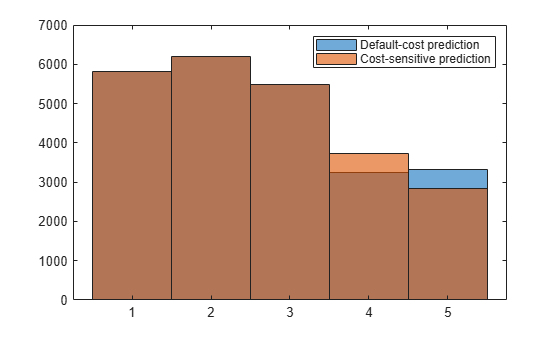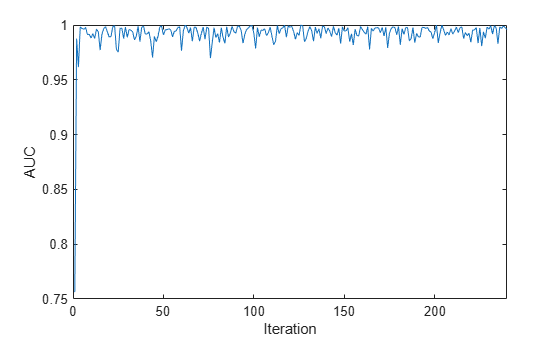predict

Predict responses for new observations from naive Bayes classification model for incremental learning

Description

example

label = predict(Mdl,X) returns the predicted responses or labels label of the observations in the predictor data X from the naive Bayes classification model for incremental learning Mdl.

example

label = predict(Mdl,X,Name,Value) specifies options using one or more name-value arguments. For example, you can specify a custom misclassification cost matrix (in other words, override the value Mdl.Cost) for computing predictions by specifying the Cost argument.

example

[label,Posterior,Cost] = predict(___) also returns the posterior probabilities (Posterior) and predicted (expected) misclassification costs (Cost) corresponding to the observations (rows) in X using any of the input-argument combinations in the previous syntaxes. For each observation in X, the predicted class label corresponds to the minimum expected classification cost among all classes.

Examples

collapse all

Load the human activity data set.

For details on the data set, enter Description at the command line.

Fit a naive Bayes classification model to the entire data set.

TTMdl = fitcnb(feat,actid)
TTMdl =
ClassificationNaiveBayes
ResponseName: 'Y'
CategoricalPredictors: []
ClassNames: [1 2 3 4 5]
ScoreTransform: 'none'
NumObservations: 24075
DistributionNames: {1×60 cell}
DistributionParameters: {5×60 cell}

Properties, Methods

TTMdl is a ClassificationNaiveBayes model object representing a traditionally trained model.

Convert the traditionally trained model to a naive Bayes classification model for incremental learning.

IncrementalMdl = incrementalLearner(TTMdl)
IncrementalMdl =
incrementalClassificationNaiveBayes

IsWarm: 1
Metrics: [1×2 table]
ClassNames: [1 2 3 4 5]
ScoreTransform: 'none'
DistributionNames: {1×60 cell}
DistributionParameters: {5×60 cell}

Properties, Methods

IncrementalMdl is an incrementalClassificationNaiveBayes model object prepared for incremental learning.

• The incrementalLearner function initializes the incremental learner by passing learned conditional predictor distribution parameters to it, along with other information TTMdl learned from the training data.

• IncrementalMdl is warm (IsWarm is 1), which means that incremental learning functions can start tracking performance metrics.

An incremental learner created from converting a traditionally trained model can generate predictions without further processing.

Predict class labels for all observations using both models.

ttlabels = predict(TTMdl,feat);
illables = predict(IncrementalMdl,feat);
sameLabels = sum(ttlabels ~= illables) == 0
sameLabels = logical
1

Both models predict the same labels for each observation.

This example shows how to apply misclassification costs for label prediction on incoming chunks of data, while maintaining a balanced misclassification cost for training.

Load the human activity data set. Randomly shuffle the data.

n = numel(actid);
rng(10); % For reproducibility
idx = randsample(n,n);
X = feat(idx,:);
Y = actid(idx);

Create a naive Bayes classification model for incremental learning; specify the class names. Prepare it for predict by fitting the model to the first 10 observations.

Mdl = incrementalClassificationNaiveBayes(ClassNames=unique(Y));
initobs = 10;
Mdl = fit(Mdl,X(1:initobs,:),Y(1:initobs));
canPredict = size(Mdl.DistributionParameters,1) == numel(Mdl.ClassNames)
canPredict = logical
1

Consider severely penalizing the model for misclassifying "running" (class 4). Create a cost matrix that applies 100 times the penalty for misclassifying running as compared to misclassifying any other class. Rows correspond to the true class, and columns correspond to the predicted class.

k = numel(Mdl.ClassNames);
Cost = ones(k) - eye(k);
Cost(4,:) = Cost(4,:)*100; % Penalty for misclassifying "running"
Cost
Cost = 5×5

0     1     1     1     1
1     0     1     1     1
1     1     0     1     1
100   100   100     0   100
1     1     1     1     0

Simulate a data stream, and perform the following actions on each incoming chunk of 100 observations.

1. Call predict to predict labels for each observation in the incoming chunk of data.

2. Call predict again, but specify the misclassification costs by using the Cost argument.

3. Call fit to fit the model to the incoming chunk. Overwrite the previous incremental model with a new one fitted to the incoming observation.

numObsPerChunk = 100;
nchunk = ceil((n - initobs)/numObsPerChunk);
labels = zeros(n,1);
cslabels = zeros(n,1);
cst = zeros(n,5);
cscst = zeros(n,5);

% Incremental learning
for j = 1:nchunk
ibegin = min(n,numObsPerChunk*(j-1) + 1 + initobs);
iend   = min(n,numObsPerChunk*j + initobs);
idx = ibegin:iend;
[labels(idx),~,cst(idx,:)] = predict(Mdl,X(idx,:));
[cslabels(idx),~,cscst(idx,:)] = predict(Mdl,X(idx,:),Cost=Cost);
Mdl = fit(Mdl,X(idx,:),Y(idx));
end
labels = labels((initobs + 1):end);
cslabels = cslabels((initobs + 1):end);

Compare the predicted class distributions between the prediction methods by plotting histograms.

figure;
histogram(labels);
hold on
histogram(cslabels);
legend(["Default-cost prediction" "Cost-sensitive prediction"])Because the cost-sensitive prediction method penalizes misclassifying class 4 so severely, more predictions into class 4 result as compared to the prediction method that uses the default, balanced cost.

Load the human activity data set. Randomly shuffle the data.

n = numel(actid);
rng(10); % For reproducibility
idx = randsample(n,n);
X = feat(idx,:);
Y = actid(idx);

For details on the data set, enter Description at the command line.

Create a naive Bayes classification model for incremental learning; specify the class names. Prepare it for predict by fitting the model to the first 10 observations.

Mdl = incrementalClassificationNaiveBayes('ClassNames',unique(Y));
initobs = 10;
Mdl = fit(Mdl,X(1:initobs,:),Y(1:initobs));
canPredict = size(Mdl.DistributionParameters,1) == numel(Mdl.ClassNames)
canPredict = logical
1

Mdl is an incrementalClassificationNaiveBayes model. All its properties are read-only. The model is configured to generate predictions.

Simulate a data stream, and perform the following actions on each incoming chunk of 100 observations.

1. Call predict to compute class posterior probabilities for each observation in the incoming chunk of data.

2. Consider incrementally measuring how well the model predicts whether a subject is dancing (Y is 5). You can accomplish this by computing the AUC of an ROC curve created by passing, for each observation in the chunk, the difference between the posterior probability of class 5 and the maximum posterior probability among the other classes to perfcurve.

3. Call fit to fit the model to the incoming chunk. Overwrite the previous incremental model with a new one fitted to the incoming observation.

numObsPerChunk = 100;
nchunk = floor((n - initobs)/numObsPerChunk) - 1;
Posterior = zeros(nchunk,numel(Mdl.ClassNames));
auc = zeros(nchunk,1);
classauc = 5;

% Incremental learning
for j = 1:nchunk
ibegin = min(n,numObsPerChunk*(j-1) + 1 + initobs);
iend   = min(n,numObsPerChunk*j + initobs);
idx = ibegin:iend;
[~,Posterior(idx,:)] = predict(Mdl,X(idx,:));
diffscore = Posterior(idx,classauc) - max(Posterior(idx,setdiff(Mdl.ClassNames,classauc)),[],2);
[~,~,~,auc(j)] = perfcurve(Y(idx),diffscore,Mdl.ClassNames(classauc));
Mdl = fit(Mdl,X(idx,:),Y(idx));
end

Mdl is an incrementalClassificationNaiveBayes model object trained on all the data in the stream.

Plot the AUC on the incoming chunks of data.

plot(auc)
ylabel('AUC')
xlabel('Iteration')The AUC suggests that the classifier correctly predicts dancing subjects well during incremental learning.

Input Arguments

collapse all

Naive Bayes classification model for incremental learning, specified as an incrementalClassificationNaiveBayes model object. You can create Mdl directly or by converting a supported, traditionally trained machine learning model using the incrementalLearner function. For more details, see the corresponding reference page.

You must configure Mdl to predict labels for a batch of observations.

• If Mdl is a converted, traditionally trained model, you can predict labels without any modifications.

• Otherwise, Mdl.DistributionParameters must be a cell matrix with Mdl.NumPredictors > 0 columns and at least one row, where each row corresponds to each class name in Mdl.ClassNames.

Batch of predictor data for which to predict labels, specified as an n-by-Mdl.NumPredictors floating-point matrix.

The length of the observation labels Y and the number of observations in X must be equal; Y(j) is the label of observation j (row or column) in X.

Data Types: single | double

Name-Value Arguments

Specify optional pairs of arguments as Name1=Value1,...,NameN=ValueN, where Name is the argument name and Value is the corresponding value. Name-value arguments must appear after other arguments, but the order of the pairs does not matter.

Example: Cost=[0 2;1 0] attributes double the penalty for misclassifying observations with true class Mdl.ClassNames(1), than for misclassifying observations with true class Mdl.ClassNames(2).

Cost of misclassifying an observation, specified as a value in the table, where c is the number of classes in Mdl.ClassNames. The specified value overrides the value of Mdl.Cost.

ValueDescription
c-by-c numeric matrix

Cost(i,j) is the cost of classifying an observation into class j when its true class is i, for classes Mdl.ClassNames(i) and Mdl.ClassNames(j). In other words, the rows correspond to the true class and the columns correspond to the predicted class. For example, Cost = [0 2;1 0] applies double the penalty for misclassifying Mdl.ClassNames(1) than for misclassifying Mdl.ClassNames(2).

Structure array

A structure array having two fields:

• ClassNames containing the class names, the same value as Mdl.ClassNames

• ClassificationCosts containing the cost matrix, as previously described.

Example: Cost=struct('ClassNames',Mdl.ClassNames,'ClassificationCosts',[0 2; 1 0])

Data Types: single | double | struct

Prior class probabilities, specified as a value in this numeric vector. Prior has the same length as the number of classes in Mdl.ClassNames, and the order of the elements corresponds to the class order in Mdl.ClassNames. predict normalizes the vector so that the sum of the result is 1.

The specified value overrides the value of Mdl.Prior.

Data Types: single | double

Score transformation function describing how incremental learning functions transform raw response values, specified as a character vector, string scalar, or function handle. The specified value overrides the value of Mdl.ScoreTransform.

This table describes the available built-in functions for score transformation.

ValueDescription
"doublelogit"1/(1 + e–2x)
"invlogit"log(x / (1 – x))
"ismax"Sets the score for the class with the largest score to 1, and sets the scores for all other classes to 0
"logit"1/(1 + ex)
"none" or "identity"x (no transformation)
"sign"–1 for x < 0
0 for x = 0
1 for x > 0
"symmetric"2x – 1
"symmetricismax"Sets the score for the class with the largest score to 1, and sets the scores for all other classes to –1
"symmetriclogit"2/(1 + ex) – 1

Data Types: char | string

Output Arguments

collapse all

Predicted responses (or labels), returned as a categorical or character array; floating-point, logical, or string vector; or cell array of character vectors with n rows. n is the number of observations in X, and label(j) is the predicted response for observation j.

label has the same data type as the class names stored in Mdl.ClassNames. (The software treats string arrays as cell arrays of character vectors.)

Class posterior probabilities, returned as an n-by-2 floating-point matrix. Posterior(j,k) is the posterior probability that observation j is in class k. Mdl.ClassNames specifies the order of the classes.

Expected misclassification costs, returned as an n-by-numel(Mdl.ClassNames) floating-point matrix.

Cost(j,k) is the expected misclassification cost of the observation in row j of X predicted into class k (Mdl.ClassNames(k)).

collapse all

Misclassification Cost

A misclassification cost is the relative severity of a classifier labeling an observation into the wrong class.

There are two types of misclassification costs: true and expected. Let K be the number of classes.

• True misclassification cost — A K-by-K matrix, where element (i,j) indicates the misclassification cost of predicting an observation into class j if its true class is i. The software stores the misclassification cost in the property Mdl.Cost, and uses it in computations. By default, Mdl.Cost(i,j) = 1 if ij, and Mdl.Cost(i,j) = 0 if i = j. In other words, the cost is 0 for correct classification and 1 for any incorrect classification.

• Expected misclassification cost — A K-dimensional vector, where element k is the weighted average misclassification cost of classifying an observation into class k, weighted by the class posterior probabilities.

${c}_{k}=\sum _{j=1}^{K}\stackrel{^}{P}\left(Y=j|{x}_{1},...,{x}_{P}\right)Cos{t}_{jk}.$

In other words, the software classifies observations to the class corresponding with the lowest expected misclassification cost.

Posterior Probability

The posterior probability is the probability that an observation belongs in a particular class, given the data.

For naive Bayes, the posterior probability that a classification is k for a given observation (x1,...,xP) is

$\stackrel{^}{P}\left(Y=k|{x}_{1},..,{x}_{P}\right)=\frac{P\left({X}_{1},...,{X}_{P}|y=k\right)\pi \left(Y=k\right)}{P\left({X}_{1},...,{X}_{P}\right)},$

where:

• $P\left({X}_{1},...,{X}_{P}|y=k\right)$ is the conditional joint density of the predictors given they are in class k. Mdl.DistributionNames stores the distribution names of the predictors.

• π(Y = k) is the class prior probability distribution. Mdl.Prior stores the prior distribution.

• $P\left({X}_{1},..,{X}_{P}\right)$ is the joint density of the predictors. The classes are discrete, so $P\left({X}_{1},...,{X}_{P}\right)=\sum _{k=1}^{K}P\left({X}_{1},...,{X}_{P}|y=k\right)\pi \left(Y=k\right).$